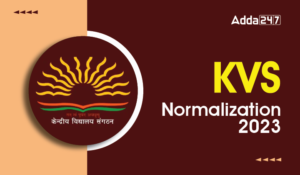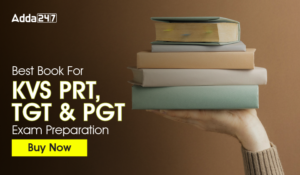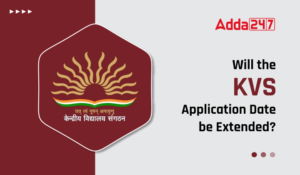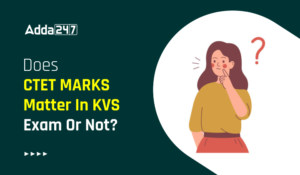Latest Teaching jobs   »   Mathematics Questions For All Teaching Exams:20th...

# Mathematics Questions For All Teaching Exams:20th July 2019

Dear Students!!! There is most general as well as a scoring section in all the competitive entrance examinations in the teaching field i.e “Mathematics”.Because in this section only one thing is work i.e your accuracy and that could be nourished with the daily practice. So, for this, we are providing you the daily quiz for all teaching exams i.e CTET Exam 2019DSSSB ,KVS,STET Exam.
Q1.The length of a string is 8 feet and 9 inches, which is divided into 3 equal parts. What is the length of each part in inches?
(a) 31
(b) 33
(c) 35
(d) 37
Q2.Determine the largest 4 digit number which is a perfect square.
(a) 9999
(b) 9702
(c) 9604
(d) 9801
Q3.Shaan got a total of Rs. 912 in the denomination of equal numbers of Rs. 1, Rs. 5 and Rs. 10 coins. How many coins do Shaan posses?
(a) 16
(b) 57
(c) 171
(d) 323
Q4.Two whole numbers are such that the square of first number exceeds the second by 112 and the ratio of the numbers is 4:3. What is the value of smaller number?
(a) 3
(b) 4
(c) 12
(d) 36

Q5.Choose the CORRECT option about the two tangents drawn to the circle from the external point?
(A) The tangents subtend equal angles at the centre of the circle.
(B) The tangents drawn from the external point to a circle are equal in length.
(C) The tangents are equally inclined to the segment which joins the centre to that point.
(a) A and B only
(b) B and C only
(c) A, B and C
(d) A and C only
Q6.Consider the figure shown below which consists of the triangle ABC which touches the circle with centre at O. Which of the following options is CORRECT?
(a) AB – CQ = AC + BQ
(b) AB + CQ = AC + BQ
(c) AB + CQ = AC – BQ
(d) None of the above
Q7.If price of oranges is increased by 30%, then a person can buy 12 oranges less for Rs 208. What is the original price (in Rs) of an orange?
(a) 2
(b) 4
(c) 8
(d) 3
Q8.Of three positive numbers, the ratio of first and second is 5 : 2, ratio of second and third is 5 : 4. The product of first and third is 1800. What is the sum of the three numbers?
(a) 43
(b) 133
(c) 119
(d) 129
Q9.The ratio of milk and water in three samples is 1 : 3, 3 : 5 and 11 : 5. A mixture containing equal quantities of all three samples is made. What will be the ratio of milk and water in the new mixture?
(a) 7 : 9
(b) 5 : 7
(c) 15 : 13
(d) 9 : 11
Q10.Average of 31 numbers is 19. If the average of first 8 numbers is 24, then what is the average of the remaining numbers?
(a) 17.26
(b) 23.25
(c) 26.2
(d) 25.45
You may also like to read :

Sharing is caring!

Thank You, Your details have been submitted we will get back to you.

### TOPICS:

•KVS Normalisation 2023, Check Process of...
•KVS Exam Centers List 2023 for TGT PGT P...
•Best Book For KVS PRT, TGT & PGT Exa...
•Will the KVS Application Date be Extende...
•Does CTET Marks Matter In KVS Exam Or No...
•Staff Shortage Affects KVs: Teaching Vac...